# Inductor Current Measurement

2016.10.06

・Inductors are important components for DC-DC converters. Evaluations are of course an indispensable part of the design process.

・In measurements an oscilloscope and a current probe are used. Confirm that the current waveform is suitable and is not saturated.

・The inductor current is examined using both peak values and average values.

The fourth topic on [Evaluating a Switching Regulator] is titled [Inductor Current Measurement], and explains the method for measuring inductor currents and important points in performing evaluations.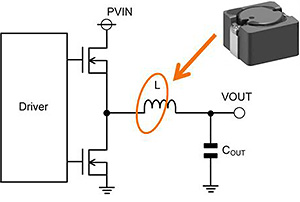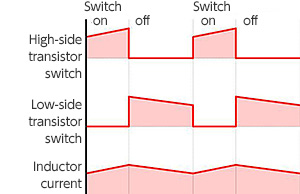### ・Regarding inductors

We begin with a slight digression. We shall here uniformly use the term “inductor” for the component marked L in the diagram on the right, used for the output of a DC-DC converter. I think it has usually been called an inductor for some time, but terms such as “coil” or “choke coil” are also frequently used. And although not heard very often, the term “reactor” is also sometimes used.

Thus in a DC-DC converter, a “coil” is a general term for a wound wire, and means the same thing as “inductor”. 　A “choke coil” is a coil for applications in which high-frequency currents are not passed. However, it seems that the differences in these terms are not very rigorously defined even within the industry, and people use terms that they are used to. I have heard (but have not confirmed the details) that “inductor” is used in the IEC and other international standards. Perhaps it is for this reason that “inductor” is coming to be used more often in recent years.

We now turn to the main topic. We begin by verifying the work done by a inductor. Put simply, a resistor limits current uniformly regardless of whether it is AC or DC, that is, regardless of the frequency, but for a given voltage, an inductor can be thought of as making current increasingly harder to flow at higher frequencies, and having no resistance component at all for direct current. When compared with capacitors, we can say that inductors are the opposite, since capacitors do not pass a DC current but easily pass an AC current. The behavior of an inductor as if it were a resistor is due to the principle of electromagnetic induction. Moreover, an inductor generates an electromotive force through self-induction. The magnitude of this effect is represented by the inductance.

In a step-down DC-DC converter, the inductor illustrated above basically has the role of smoothing the output. When an on/off square-wave voltage is input by an output transistor to the inductor shown above, the flowing current is converted by the inductor into a sawtooth waveform as a gradient is formed in the in- and outflowing current. In this way, a square wave is smoothed into a sawtooth wave. This is the essence of the inductor action.

### ・Inductor current and output ripple voltage

In order to measure an inductor current and judge whether it is suitable, the inductor current and output ripple voltage are represented by equations. (In the diagram on the lower-left, the word “coil” is used owing to the origin of the diagram; of course this is the inductor.)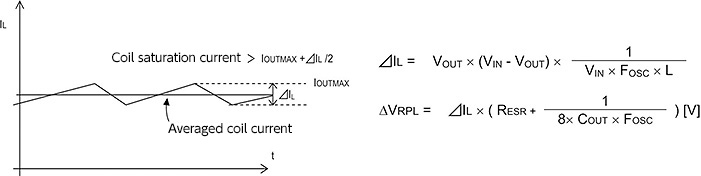These equations always arise when designing a DC-DC converter. Evaluations are probably made easier if the relations of the terms in these equations can be visualized.

The peaks of the sawtooth-wave inductor current, represented by ΔIL, become smaller as the magnitude of the inductance L is increased. We see that they also become smaller as the switching frequency is raised. We see that the ripple voltage, represented by ΔVREP, is dominated by ΔIL and the capacitor ESR. Based on this, the measured inductor current is evaluated.

### ・Method of measuring the inductor current

The inductor current waveform is observed using an oscilloscope and a current probe. The current probe must clamp the current path, and so a wire onto which the probe is clipped must be exposed, as in the photo.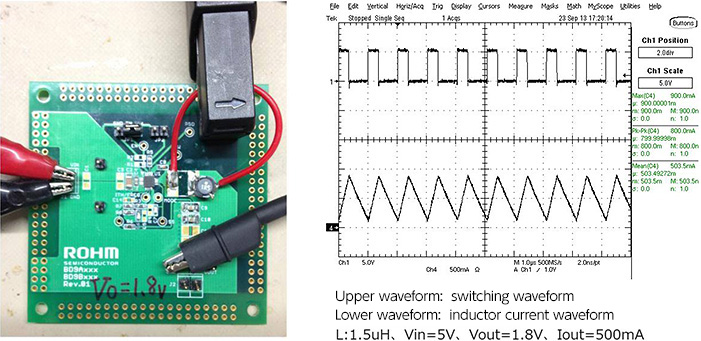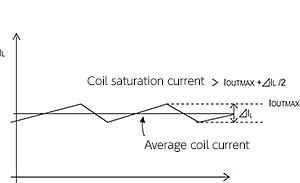### ・Consideration on inductor current

In the waveform graph on the upper right, the upper waveform is a switching voltage waveform, and the lower waveform is an inductor current waveform. These actually observed waveforms can be said to be close to ideal, clean sawtooth waveforms.

Important points in waveform evaluation are explained using the diagram on the right already introduced. These are points used at design time in order to determine the inductor specifications. That is, it is in effect necessary to confirm that the inductor selected during design is actually appropriate.

The inductor (coil) should be selected such that the saturation current of the inductor is greater than the value of IOTMAX added to ΔIL/2. It should be compared with the measured value.

If the allowed saturation current value is inadequate, the inductor reaches saturation and a current flows suddenly, and the gradient of the sawtooth waveform is distorted into a quadratic curve. In the worst case, a current greater than that presumed may flow in the switching transistor, resulting in failure. This is an important point to check.

### Points in evaluating inductor currents

Important points when evaluating inductors and inductor currents are summarized below.

• Component values are determined according to data sheets, but actual measurements must always be made.
• An oscilloscope and a current probe are used in measurements.
• It must be confirmed that a current waveform is suitable and is not saturated.
• An inductor current is analyzed using both peak and averaged values.
• To select an inductor, numerical equations are used and IOUTMAX and ΔIL/2 are considered.

The behavior of an inductor is sometimes hard to grasp, and it seems that inductors are somewhat disliked for this reason. However, they are indispensable components for DC-DC converters, and a deep understanding of inductors should be gained by repeated evaluations based on actual measurements.